# PixelGAN Autoencoders

PixelGAN is an autoencoder for which the generative path is a convolutional autoregressive neural network on pixels, conditioned on a latent code, and the recognition path uses a generative adversarial network (GAN) to impose a prior distribution on the latent code.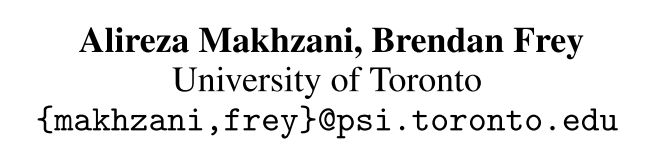## 1. Introduction

This paper proposed a “PixelGAN Autoencoder”, for which the generative path is a convolutional autoregressive neural network on pixels, conditioned on a latent code, and the recognition path uses a generative adversarial network (GAN) to impose a prior distribution on the latent code. This paper also shows different priors result in different decompositions of information between the latent code and the auto-regressive decoder.

## 2. Methods

A Quick Review of GAN
Generative Adversarial Network originally consists of one generator and one discriminator. The generator G samples the prior p(z) and generates the fake sample G(z) to maximally confuse the discriminator. The discriminator D(x) is trained to identify whether the input x is a sample from the real data distribution or a sample from the generative model. Mathematically, the cost function of GAN is defined as follows: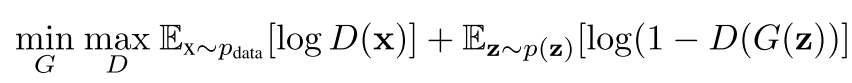PixelGAN Autoencoder
The key difference of PixelGAN Autoencoder from the previous “Adversarial Autoencoders” is that the normal deterministic decoder part of the network is replaced by a more powerful decoder — “PixelCNN”.

The recognition path of the PixelGAN autoencoder defines an implicit posterior distribution q(z|x), by using a deterministic neural function z = f (x, n) that takes the input x, along with random noise n with a fixed distribution p(n), and outputs z. The aggregated posterior q(z) of this model is defined as follows: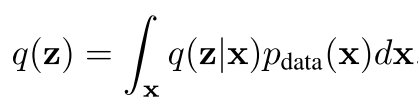Samples from this implicit distribution q(z|x) are achieved by evaluating f (x, n) as different samples of n.

The generative path p(x|z) is a conditional PixelCNN, conditioning on the latent vector z using an adaptive bias in PixelCNN layers. An adversarial network is attached on top of the hidden code vector of the autoencoder, and matches the aggregated posterior distribution q(z) to an arbitrary prior p(z).

Thus, the Architecture of this PixelGAN Autoencoder is shown in figure 1 as follows:Training Method

The adversarial network, the PixelCNN decoder and the encoder are trained jointly in two phases – the reconstruction phase and the adversarial phase – executed on each mini-batch.

In the reconstruction phase, the input x (ground truth) along with the latent vector z inferred by the encoder are provided to the PixelCNN decoder. The PixelCNN decoder weights are updated to maximize the log-likelihood of the input x. The encoder weights are updated at this stage by the gradient that comes through the conditioning vector of the PixelCNN.

In the adversarial phase, the adversarial network updates both its discriminative network and its generative network (the encoder) to match q(z) to p(z).

The connection between the PixelGAN Autoencoder cost and maximum likelihood learning is established by using a decomposition of the aggregated evidence lower bound (ELBO):In equation (2), the second term is the marginal KL divergence between the aggregated posterior and the prior distribution. The third term is the mutual information between latent vector z and input x, which works as a regularization term to encourage z and x to be decoupled.

In order to obtain a more useful/meaningful representation, the authors modify the ELBO by removing the mutual information term, since this term favours z to be independent of x. A meaningful representation here means that z is highly dependent of x. In other words, the latent vectors can extract some hidden structures from the training data, such that it is possible to generate more realistic data by sampling from the latent distribution after training.

## 3. Experiments and ResultsFigure 2 shows that PixelGAN Autoencoder with Gaussian priors can decompose the global and local statistics of the images between the latent code and the autoregressive decode: Sub-figure 2(a) shows that the samples generated from PixelGAN have sharp edges with global statistics (it is possible to recognize the number from these samples). But with the receptive field of the same size, the PixelCNN can only learn the local statistics (sharp edges in the samples), but fails to capture the global statistics as shown in sub-figure 2(b), because the receptive field is too small for PixelCNN. Sub-figure 2(c) shows that Adversarial Autoencoder (AAE) is able to capture the global statistics, but generates samples with blurry edges.Figure 4 shows that the authors use the categorical prior to impose the q(z), and show that this PixelGAN Autoencoder can separate the discrete information (categorical prior here) from the continuous information in the images.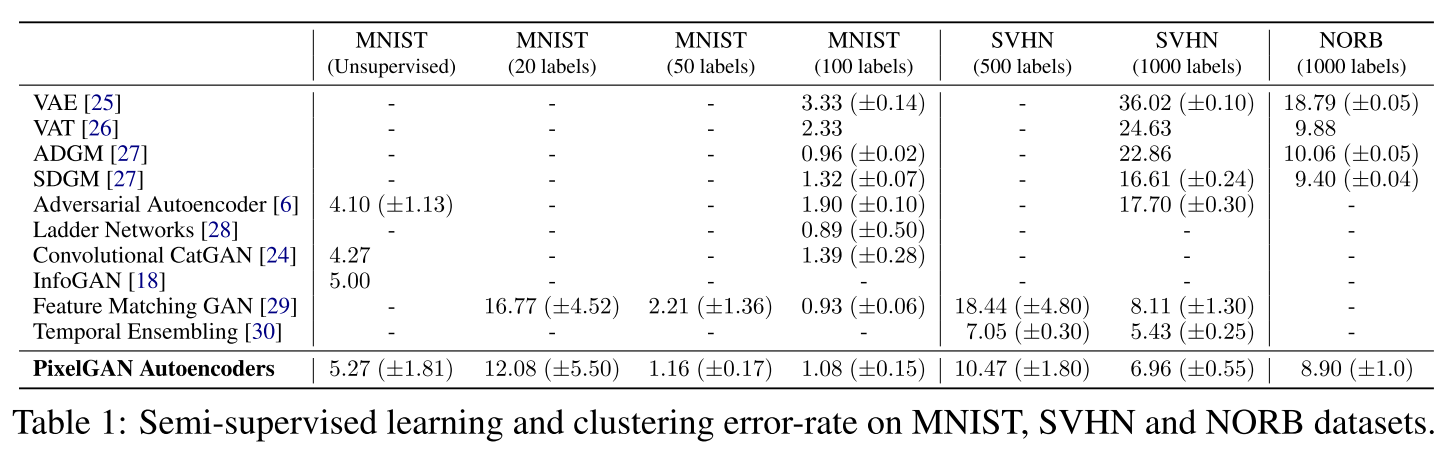Table 1 shows the performance of PixelGAN Autoencoder is on par with other GAN-based clustering algorithms like CatGAN, InfoGAN and adversarial autoencoder. For the MNIST test, the performance of the PixelGAN is highly competitive to other methods, and the performance is better when the training data has more labels. For the SVHN 500 and 1000 labels test, the PixelGAN performs better than any other generative models shown in table 1. Only “Temporal Ensembling”, which is not a generative model, has a better result than PixelGAN. For the NORB test, PixelGAN outperforms all the other reported results.

For unsupervised clustering, they use the following evaluation metric:

Once the training is done, for each cluster i, they found the validation example x_n that maximizes q(z_i|x_n), and assigned the label of x_n to all the points in the cluster i. They then computed the test error based on the assigned class labels to each cluster.

The visual results are as follows:## 4. Conclusion

The authors present the PixelGAN Autoencoder. They show that by imposing a Gaussian prior, the local and global statistics of the images are able to be disentangled, and by imposing a categorical prior, the style and content of images are able to be disentangled. They also demonstrate the application of the PixelGAN Autoencoder in downstream tasks such as semi-supervised learning.

## 5. Thoughts from the Reviewer

This work is more like an enhanced version of “Adversarial Autoencoder” (AAE) proposed by the same authors in paper Adversarial Autoencoders . The architecture of AAE is as follows: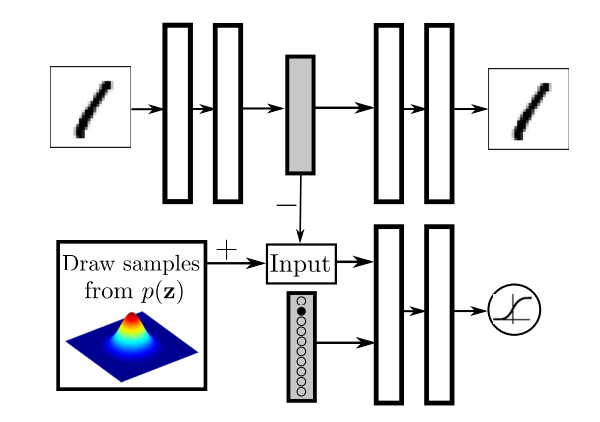The main character of this architecture is that the adversarial game is designed between a given prior and a latent vector encoded by autoencoder. Compared to this, the previous GANs are trained to distinguish the real images from fake images, rather than their embedding or latent vector representations. Thus, AAE has the advantage that it is able to capture the meaningful information from training data by imposing a prior on the latent vector.

This paper keeps this advantage and modifies the architecture as follows: The normal decoder part of a conventional autoencoder is replaced by PixelCNN proposed in paper Conditional Image Generation with PixelCNN Decoders .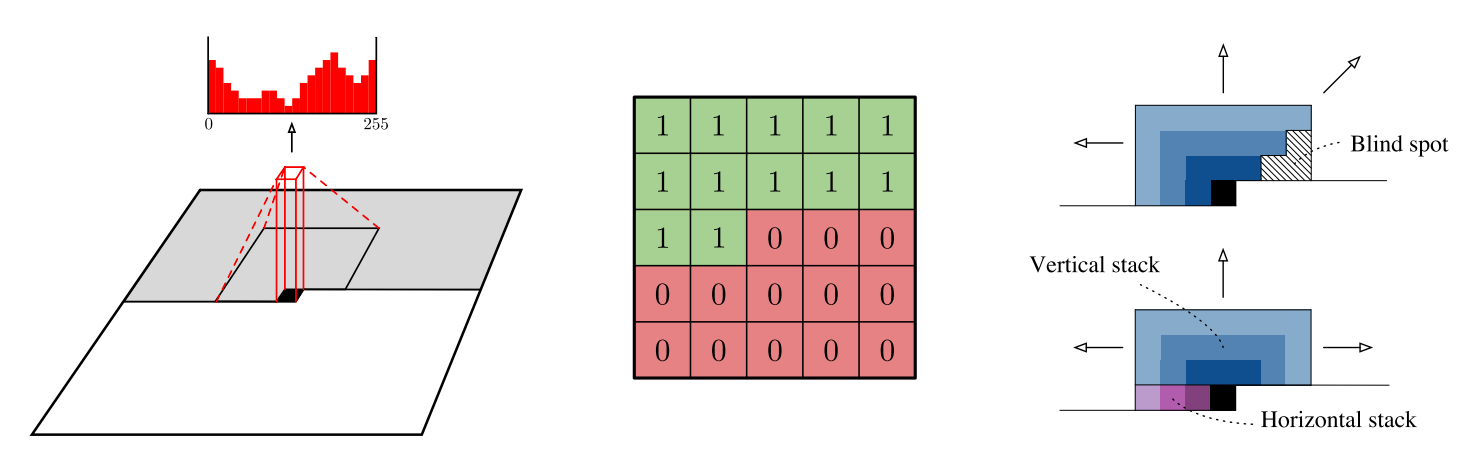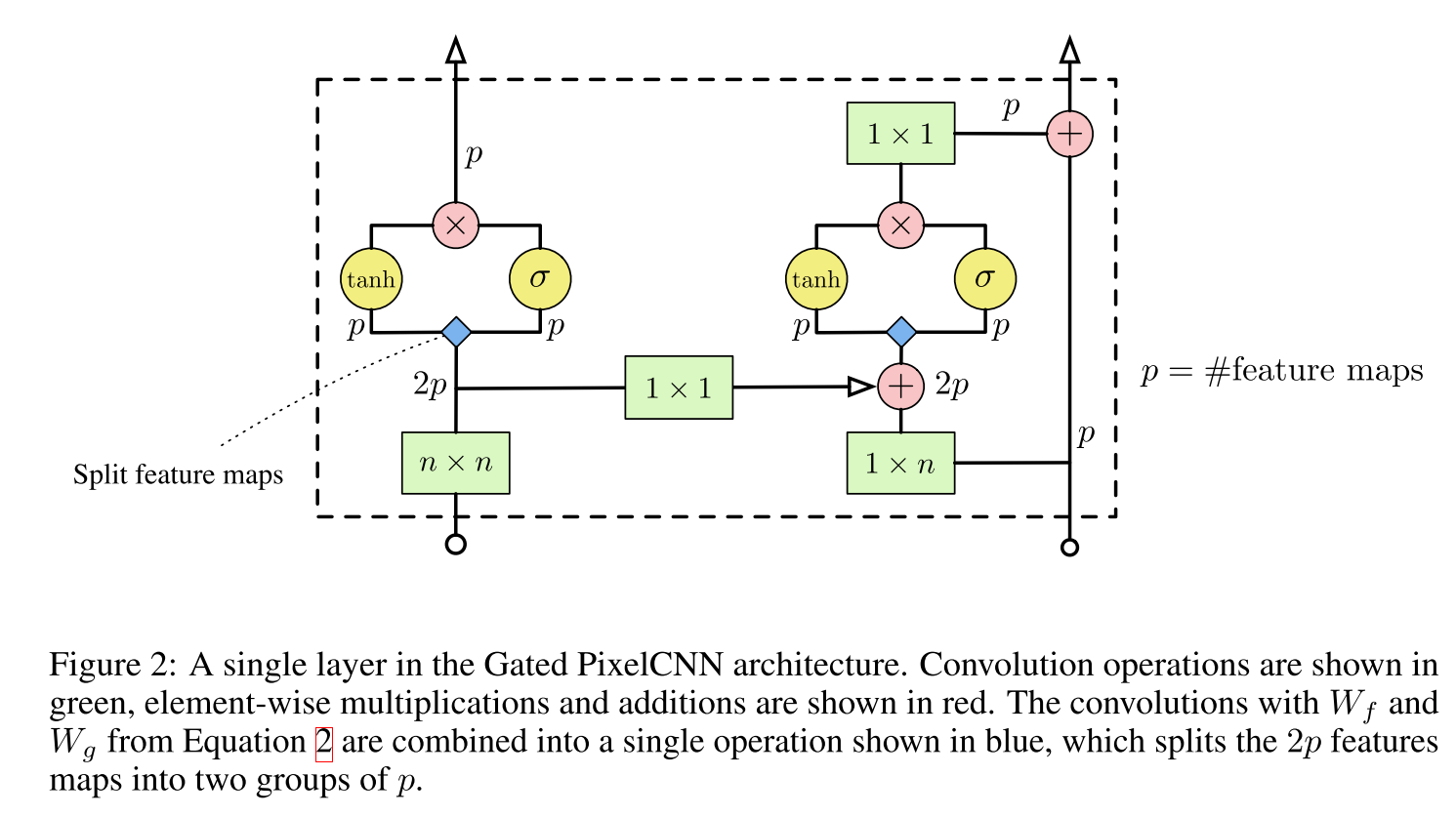As shown in the figures above, PixelCNN is a very powerful decoder which can catch very low-level information between pixels. Hence, this PixelGAN Autoencoder is not only able to capture high-level information (global statistics) but also to learn the low-level informations (local statistics).

Paper Source: https://arxiv.org/pdf/1706.00531.pdf

Reference:

Author: Liao | Technical Reviewer: Haojin Yang